## Example Questions

### Example Question #41 : Equations / Inequalities

A theme park charges $10 for adults and$5 for kids. How many kids tickets were sold if a total of 548 tickets were sold for a total of $3750? Possible Answers: 157 269 248 431 346 Correct answer: 346 Explanation: Let c = number of kids tickets sold. Then (548 – c) adult tickets were sold. The revenue from kids tickets is$5c, and the total revenue from adult tickets is $10(548 – c). Then, 5c + 10(548 – c) = 3750 5c + 5480 – 10c = 3750 5c = 1730 c = 346. We can check to make sure that this number is correct:$5 * 346 tickets + $10 * (548 – 346) tickets =$3750 total revenue

### Example Question #44 : Equations / Inequalities

Two palm trees grow next to each other in Luke's backyard. One of the trees gets sick, so Luke cuts off the top 3 feet. The other tree, however, is healthy and grows 2 feet. How tall are the two trees if the healthy tree is now 4 feet taller than the sick tree, and together they are 28 feet tall?

11 and 17 feet

cannot be determined

12 and 16 feet

14 and 14 feet

8 and 20 feet

12 and 16 feet

Explanation:

Let s stand for the sick tree and h for the healthy tree. The beginning information about cutting the sick tree and the healthy tree growing is actually not needed to solve this problem! We know that the healthy tree is 4 feet taller than the sick tree, so h = s + 4.

We also know that the two trees are 28 feet tall together, so s + h = 28. Now we can solve for the two tree heights.

Plug h = s + 4 into the second equation: (s + 4) + s = 28. Simplify and solve for h: 2s = 24 so s = 12. Then solve for h: h = s + 4 = 12 + 4 = 16.

### Example Question #41 : Linear / Rational / Variable Equations

Solve for z

3(z + 4)3 – 7 = 17

4

2

–8

–2

8

–2

Explanation:

1. Add 7 to both sides

3(z + 4)3 – 7 + 7= 17 + 7

3(z + 4)3 = 24

2. Divide both sides by 3

(z + 4)3 = 8

3. Take the cube root of both sides

z + 4 = 2

4. Subtract 4 from both sides

z = –2

### Example Question #81 : Algebra

Jen and Karen are travelling for the weekend. They both leave from Jen's house and meet at their destination. Jen drives 45mph the whole way. Karen drives 60mph but leaves a half hour after Jen. How long after Jen leaves does Karen catch up with her?

She can't catch up.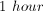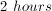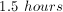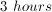Explanation:

For this type of problem, we use the formula: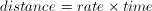When Karen catches up with Jen, their distances are equivalent. Thus,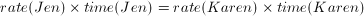We then make a variable for Jen's time,. Thus we know that Karen's time is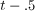(since we are working in hours).

Thus,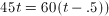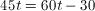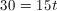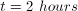### Example Question #81 : Algebra

Bill and Bob are working to build toys. Bill can build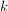toys in 6 hours. Bob can buildtoys in 3 hours. How long would it take Bob and Bill to build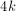toys working together?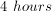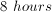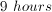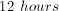Explanation:

Bill builds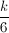toys an hour. Bob builds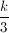toys an hour. Together, their rate of building is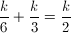. Together they can buildtoys in 2 hours. They would be able to buildtoys in 8 hours.

### Example Question #1856 : Sat Mathematics

Audrey, Penelope and Clementine are all sisters. Penelope is 8 years older than Clementine and 2 years younger than Audrey. If the sum of Penelope and Clementine's age is Audrey's age, how old is Clementine's age?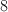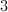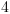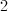Explanation:

Let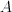= Audrey's age,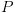= Penelope's age, and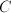= Clementine's age.

Since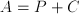, then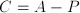.

Furthermore,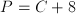, and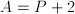.

Through substitution,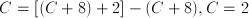.

### Example Question #1857 : Sat Mathematics

If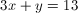and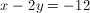, what is the value of?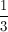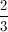Explanation:

We could use the substitution or elimination method to solve the system of equations. Here we will use the elimination method.

To solve for, combine the equations in a way that makes the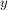terms drop out. The first equation hasand the second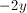, so multiplying the first equation times 2 then adding the equations will eliminate theterms.

Multiplying the first equation times 2: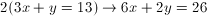Adding this result to the second equation: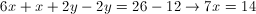Isolateby dividing both sides by 7: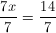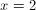### Example Question #51 : Linear / Rational / Variable Equations

If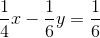and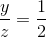, then what is the value of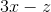?Explanation:

Since the expression we want just involves z and x, but not y, we start by solvingfor y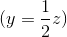.

Then we can plug that expression in for y in the first equation.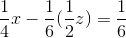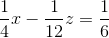Multiply everything by 12 to get rid of fractions.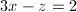### All SAT Math Resources